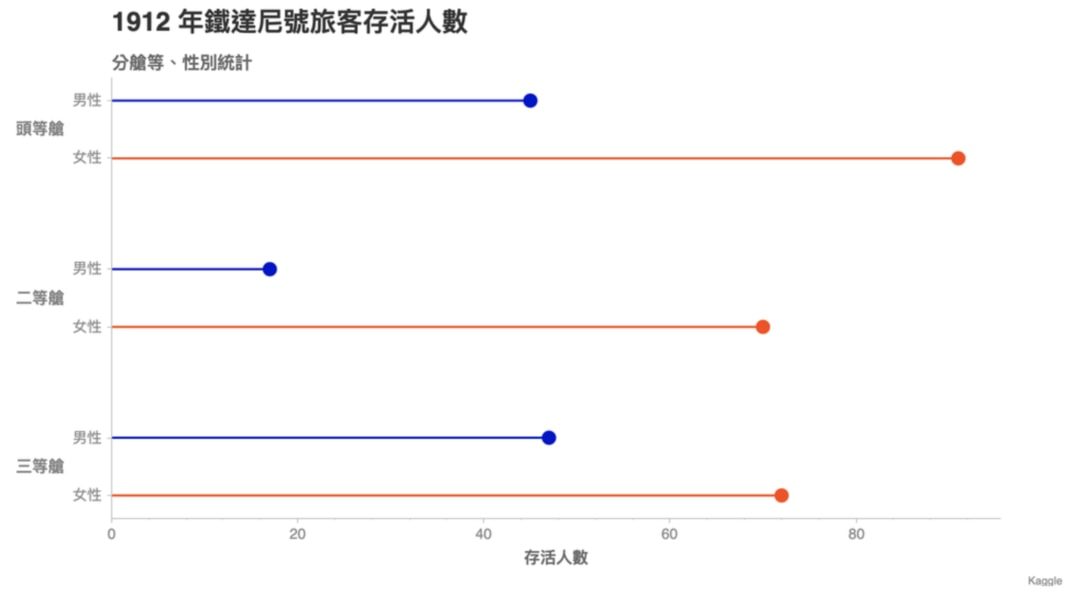## Chartify 來拯救世界¶

Spotify 最近開源了一個簡單卻同時強大的 Python 資料視覺化 Package：Chartify。我在工作中實際使用後覺得非常方便，所以想在這邊跟你分享我的經驗、並展示如何實際利用 Chartify 來視覺化一些台灣的 Open Data，讓你快速上手 Chartify。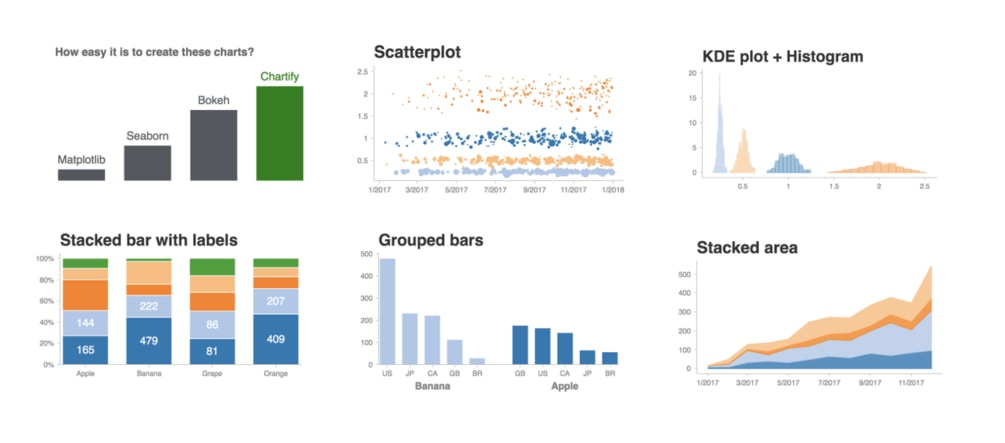Chartify 圖表 （圖片來源

Chartify 有 3 大優點：

• 預設就很漂亮的圖表樣式
• 簡單直覺的 API
• 內建非常詳細的使用說明

## 這篇適合誰¶

• 菜鳥 DS：從頭了解如何使用 Chartify 及 Python 來產生漂亮圖表
• 老手 DS：利用 Chartify 來有效率地解決你目前 80 % 的繪圖需求
• 所有想要快速學會如何使用 Python 來做資料視覺化的你## Python 函式庫¶

import pandas as pd
import chartify


## 範例資料及 Tidy 格式¶

df = chartify.examples.example_data()

date country fruit unit_price quantity total_price
0 2017-10-21 US Banana 0.303711 4 1.214846
1 2017-05-30 JP Banana 0.254109 4 1.016436
2 2017-05-21 CA Banana 0.268635 4 1.074539
3 2017-09-18 BR Grape 2.215277 2 4.430554
4 2017-12-08 US Banana 0.308337 5 1.541687

• 1 個變數只存在一欄裡頭（Column）
• 一列（Row）則代表 1 個觀測結果

df.pivot_table(
values=['unit_price'],
index='country',
columns=['fruit'])

unit_price
fruit Apple Banana Grape Orange
country
BR 0.978156 0.252695 2.022562 0.501151
CA 1.009426 0.249756 1.997512 0.504068
GB 1.007317 0.241405 2.002525 0.501009
JP 0.988600 0.249436 2.060732 0.499539
US 0.987463 0.252078 1.970634 0.504666

## 第一個 Chartify Chart¶

ch = chartify.Chart()
ch.plot.scatter(
data_frame=df,
x_column='unit_price',
y_column='total_price'
)
ch.show('png')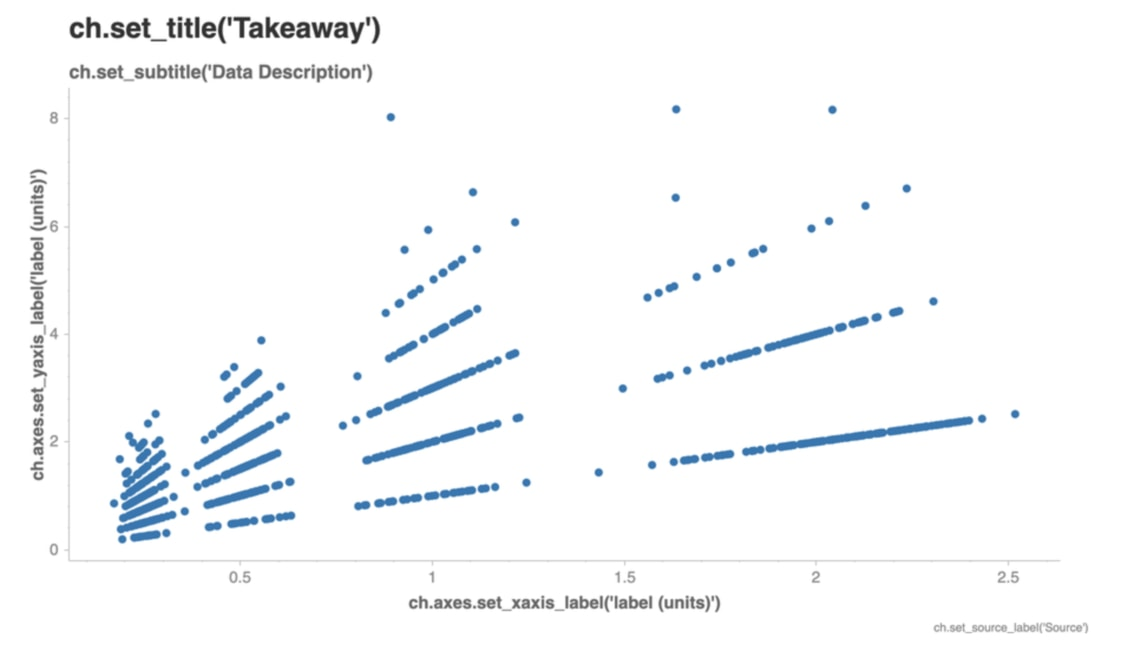ch = chartify.Chart()
ch.plot.scatter(
data_frame=df,
x_column='unit_price',
y_column='total_price')
ch.show('png')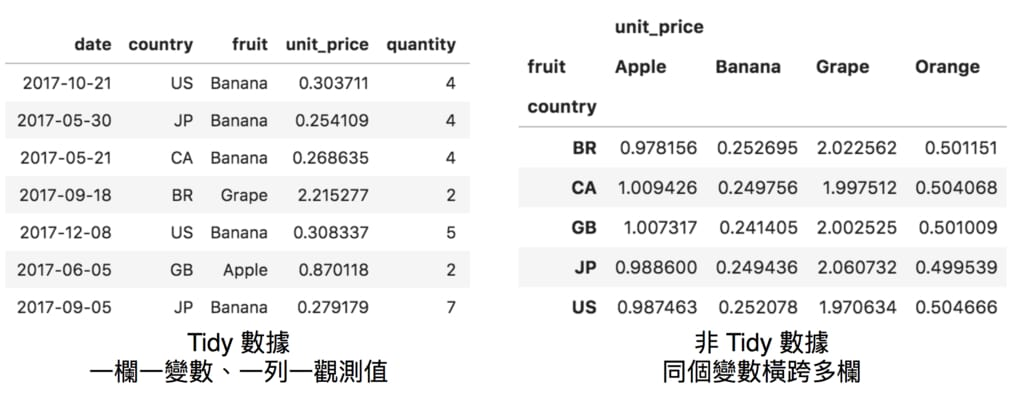## Chartify 提醒你提供重要訊息¶

• 圖表標題、副標
• X、Y 軸標籤
• 圖片的數據來源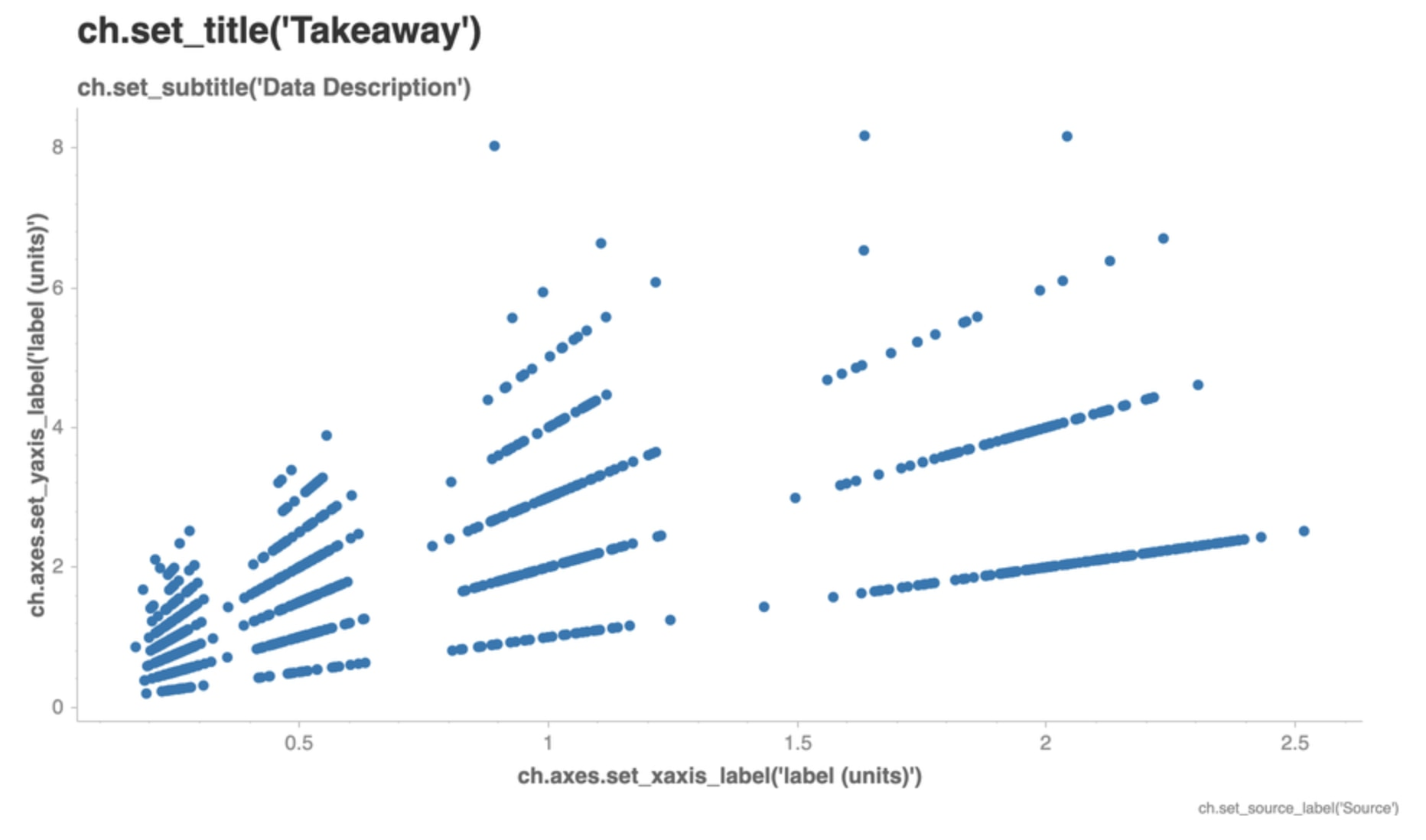ch.set_title('水果單價與總價格關係')


ch = chartify.Chart()
ch.plot.scatter(
data_frame=df,
x_column='unit_price',
y_column='total_price')

# 這部分程式碼都已經被寫在圖上
# 照抄即可：）
ch.set_title('水果單價與總價格關係')
ch.set_subtitle('Chartify 內建數據')
ch.set_source_label('Chartify')
ch.axes.set_xaxis_label('單價')
ch.axes.set_yaxis_label('總價格')

ch.show('png')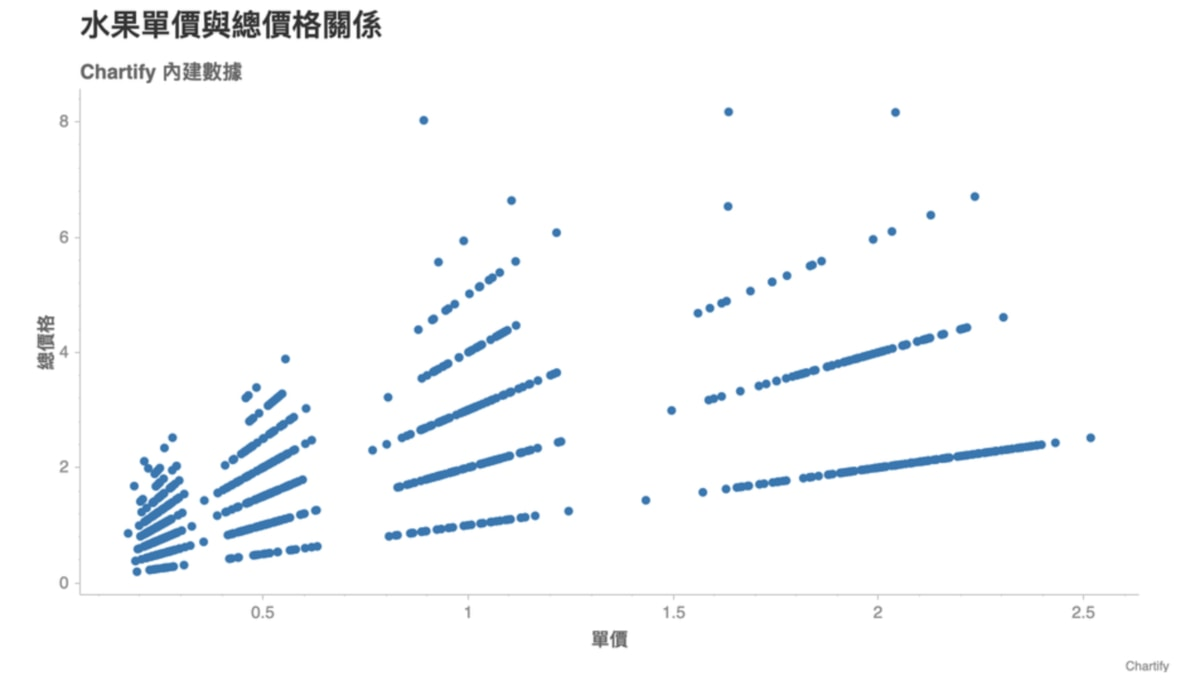## 凡事有例外，或是懶了¶

ch = chartify.Chart(
blank_labels=True)
ch.plot.scatter(
data_frame=df,
x_column='unit_price',
y_column='total_price')
ch.set_title('水果單價與總價格關係')
ch.show('png')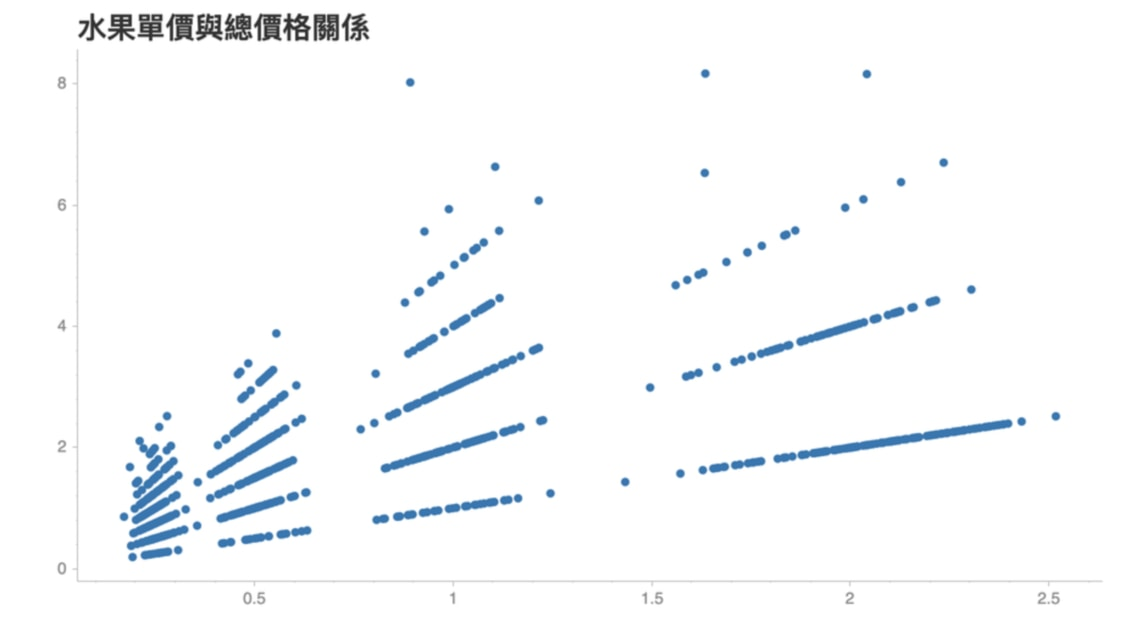## 每年有多少人拜訪台灣？¶df.head(6)

continent year visits
0 亞洲地區 2002 1689854
1 歐洲地區 2002 209110
2 美洲地區 2002 439403
3 華僑 2002 539164
4 大洋洲 2002 48334
5 非洲地區 2002 9479

ch = chartify.Chart(
x_axis_type='datetime')

ch.plot.area(
data_frame=df,
x_column='year',
y_column='visits',
color_column='continent',
stacked=True)

# 所有繪圖工具都需要這些說明
ch.set_title('歷年各大洲及華僑來台旅客人次')
ch.set_subtitle('西元 2002 至 2016 年')
ch.set_source_label('政府資料開放平臺')
ch.axes.set_xaxis_label('西元年份')
ch.axes.set_yaxis_label('來台旅客人次')

ch.show('png')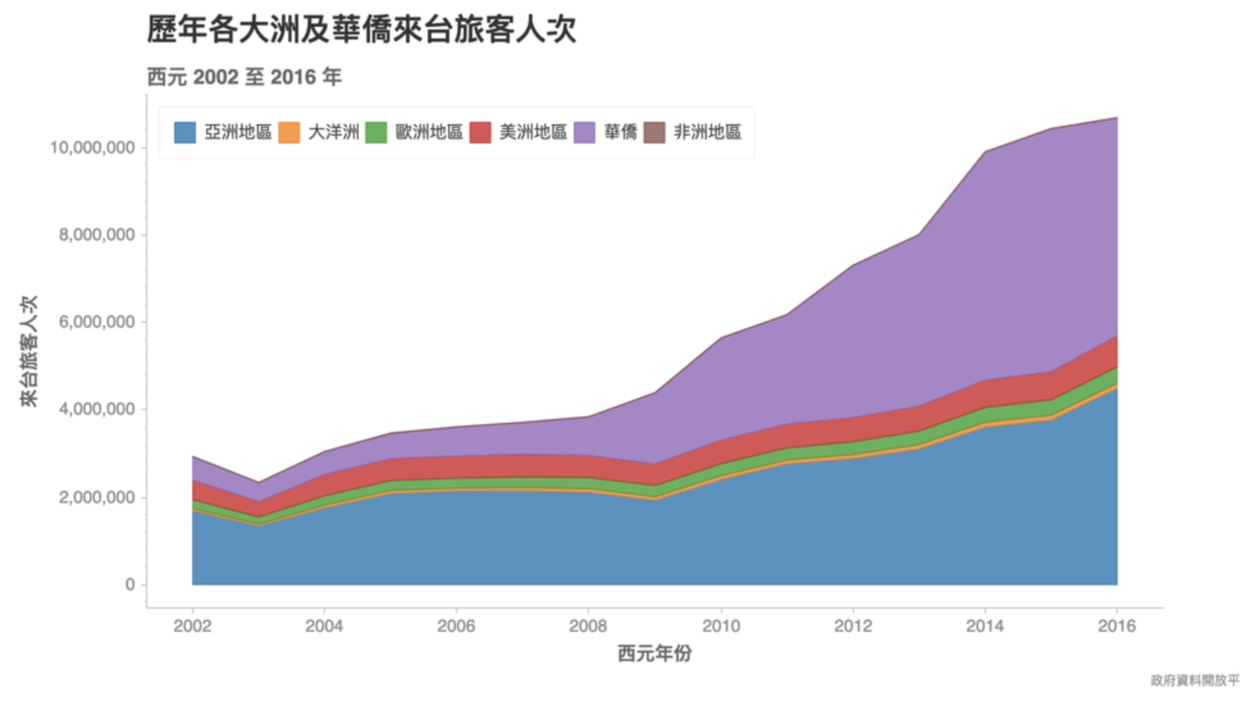ch.plot.area(
data_frame=df,
x_column='year',
y_column='visits',
color_column='continent',
stacked=True)


ch = chartify.Chart(
x_axis_type='datetime')## 一個參數就能轉變圖表¶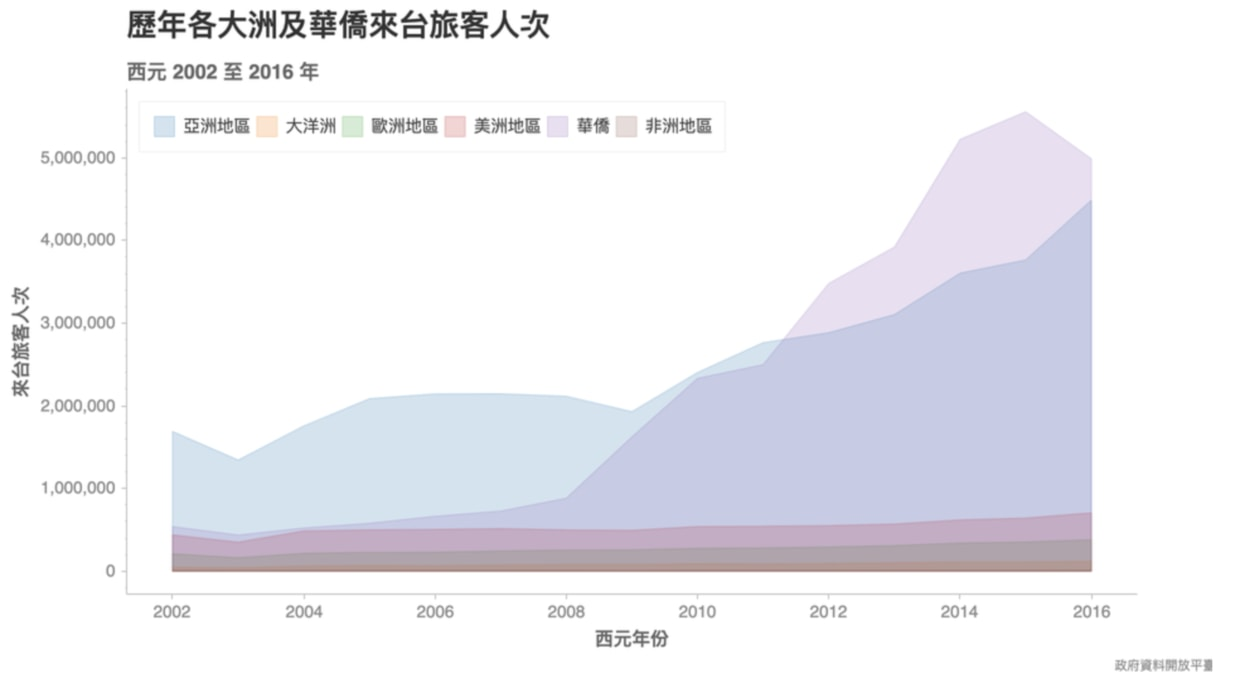## 所以大家來台灣做什麼？¶df.head(8)

continent purpose visits
0 亞洲地區 其他 1531958
1 亞洲地區 展覽 10814
2 亞洲地區 探親 221338
3 亞洲地區 會議 47428
4 亞洲地區 業務 530369
5 亞洲地區 求學 53118
6 亞洲地區 觀光 7192696
7 亞洲地區 醫療 37072

（當然，這個 DataFrame 也經過前處理，被轉換成適合繪圖的 Tidy 格式：一欄一變數）

ch = chartify.Chart(
blank_labels=True,
x_axis_type='categorical')

ch.plot.bar_stacked(
data_frame=df,
categorical_columns='continent',
stack_column='purpose',
numeric_column='visits',
normalize=True)

# 將圖例移到圖表下方，方便閱讀
ch.set_legend_location('outside_bottom')

# Chartify 支持 Chain Operation
(ch.set_title('2016 年各大洲旅客來台目的統計')
.set_subtitle('目的百分比（％）')
.axes.set_xaxis_label('各大洲')
.axes.set_yaxis_label('來台旅客目的佔比（％）')
.show('png'))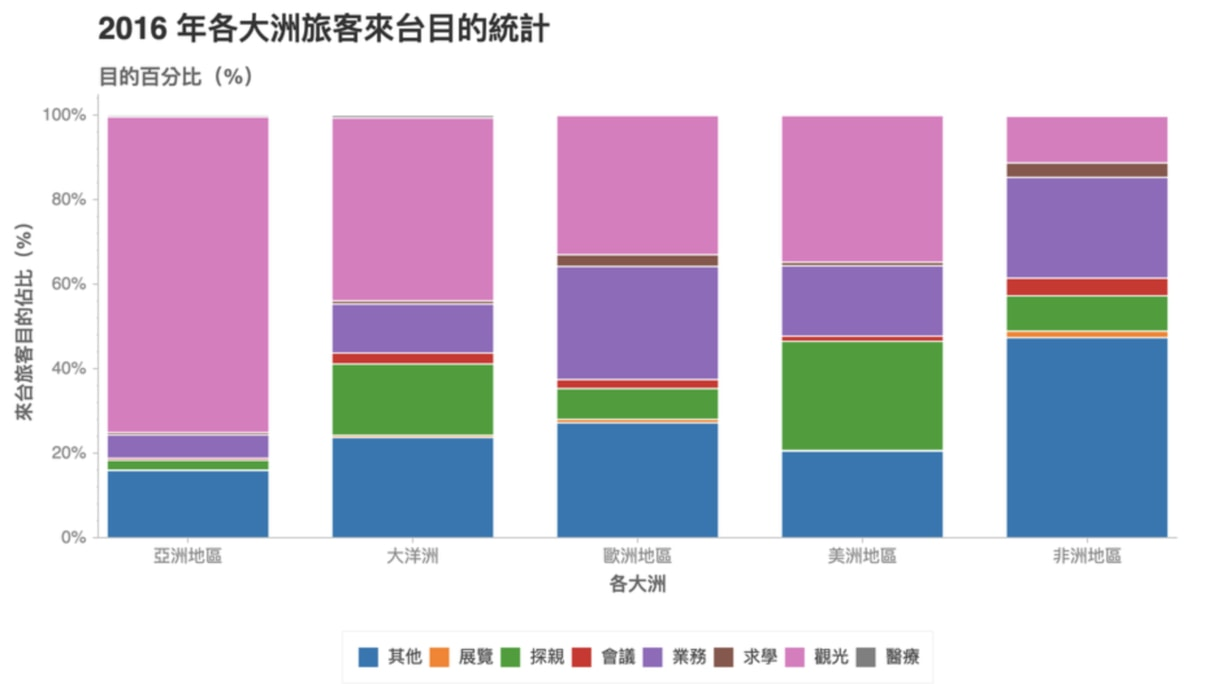• 亞洲國家超級喜歡來台灣觀光
• 歐洲及非洲的來台求學比例較其他洲高
• 美洲國家來台探親的需求意外地高
• 非洲地區的「其他」目的耐人尋味

ch = chartify.Chart(
blank_labels=True,
x_axis_type='categorical')

ch.plot.bar_stacked(
data_frame=df,
categorical_columns='continent',
stack_column='purpose',
numeric_column='visits',
normalize=True)## 一個字母就能翻轉世界¶

ch = chartify.Chart(
blank_labels=True,
x_axis_type='categorical')


ch = chartify.Chart(
blank_labels=True,
y_axis_type='categorical')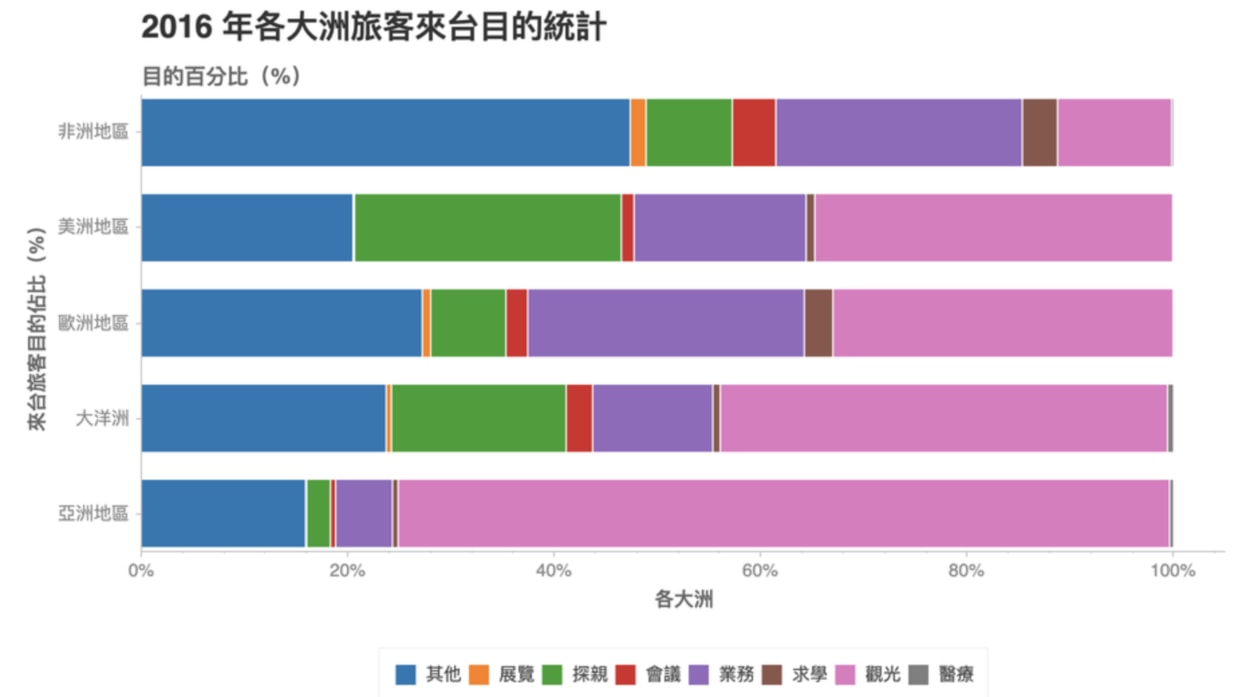## 在台北市，哪些地區比較多意外事故？¶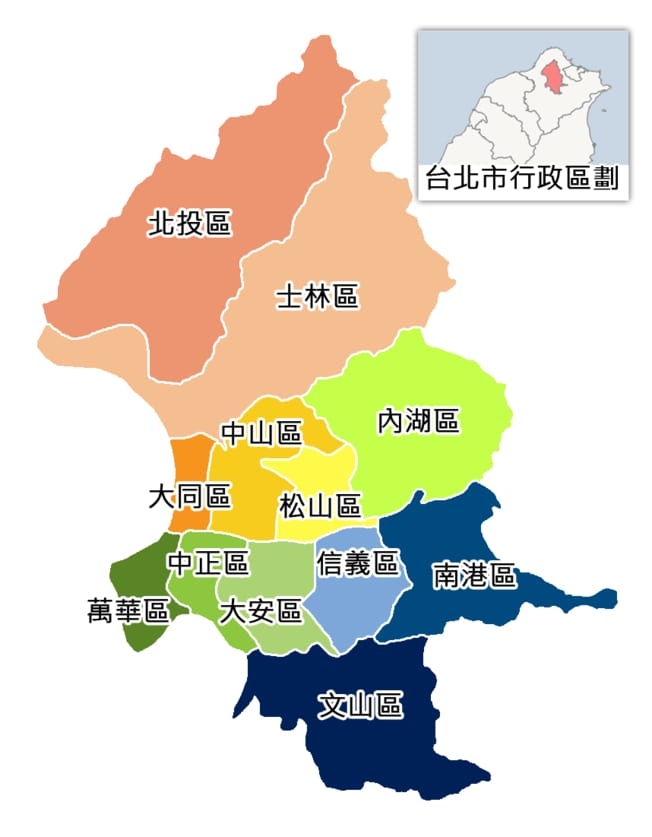df.head()

district sex injury
0 南港區 女性 799
1 萬華區 女性 1084
2 松山區 女性 1146
3 大同區 女性 1336
4 士林區 女性 1660

ch = chartify.Chart(
x_axis_type='categorical',
y_axis_type='categorical')

ch.plot.heatmap(
data_frame=df,
y_column='sex',
x_column='district',
color_column='injury',
text_column='injury',
color_palette='Reds',
text_format='{:,.0f}')

(ch.set_title('2017 年台北市各行政區交通意外受傷人數')
.set_subtitle('性別分計')
.set_source_label("Data.Taipei")
.axes.set_xaxis_label('性別')
.axes.set_yaxis_label('行政區')
.show('png'))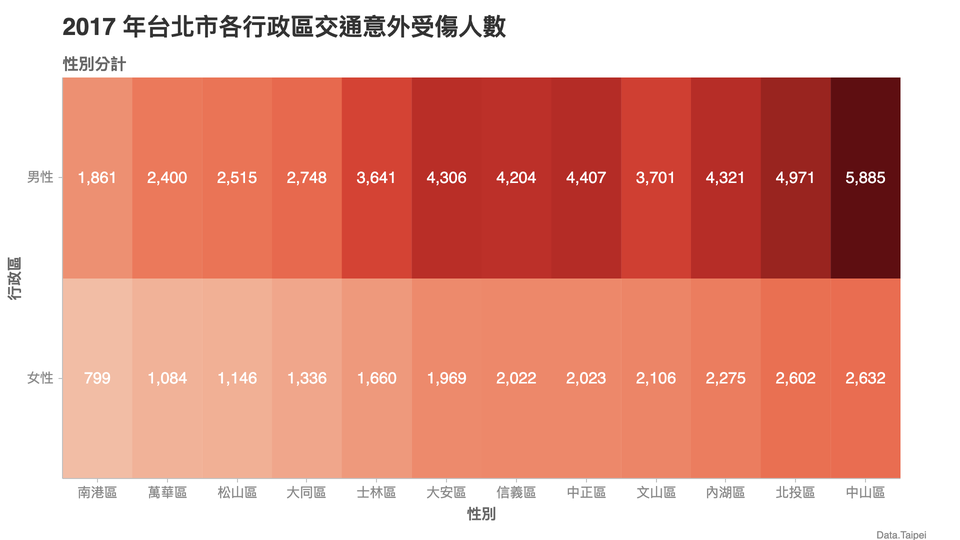## Chartify 心法及資料視覺化¶

1. 定義一個 Chart 物件
2. 決定 X、Y 軸的變數型態（如 categorical
3. 決定圖表類型（如 scatterheatmap 等）
4. 決定各個變數要對應到什麼視覺變數（如 X 軸、顏色等）
5. 加入標題與 X、Y 軸標籤
6. 渲染圖表，開心分享結果

## 結語¶• 前往 官方 Github 查看 Chartify 的安裝以及其他指南
• 利用本章學到的基礎，探索 Chartify 提供的教學 Notebook 以及瀏覽所有範例圖表
• 實際應用本文所學在自己的生活或是工作裡頭，快速提升效率
• 分享本文給可能會對 Python 或資料視覺化感興趣的朋友，讓他們感謝你

## Chartify 畫廊¶

### Lollipop Chart¶

df = pd.read_csv('dataset/titanic-train.csv')

df_group = df.groupby(['Pclass', 'Sex'])['Survived'].sum().reset_index()
df_group.Pclass.replace({1: '頭等艙', 2: '二等艙', 3: '三等艙'}, inplace=True)
df_group.Sex.replace({'female': '女性', 'male': '男性'}, inplace=True)

ch = chartify.Chart(
blank_labels=True,
y_axis_type='categorical')
ch.style.set_color_palette('categorical', ['OrangeRed', 'Mediumblue'])
ch.plot.lollipop(
data_frame=df_group,
categorical_columns=['Pclass', 'Sex'],
numeric_column='Survived',
color_column='Sex',
categorical_order_by='labels',
categorical_order_ascending=True
)

ch.set_title('1912 年鐵達尼號旅客存活人數')
ch.set_subtitle('分艙等、性別統計')
ch.set_source_label('Kaggle')
ch.axes.set_xaxis_label('存活人數')
ch.axes.set_yaxis_tick_orientation(['horizontal', 'horizontal'])
ch.show('png')